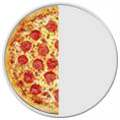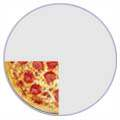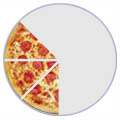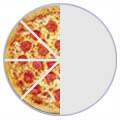# Fractions with 4W 4/3

Fractions

A fraction is a part of a whole1/2 1/4 3/8 (One-Half) (One-Quarter) (Three-Eighths)

Maths Dictionary – Equivalent Fractions

## Equivalent Fractions

Some fractions may look different, but are really the same, for example:

 4/8 = 2/4 = 1/2 (Four-Eighths) Two-Quarters) (One-Half)==It is usually best to show an answer using the simplest fraction ( 1/2 in this case ). That is called Simplifying, or Reducing the Fraction

Fraction MonkeysFraction Wall Sheet